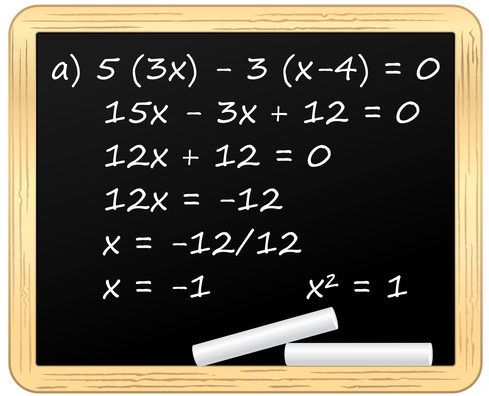Home >> Preparation Advice >> Question Types >> Problem Solving

# Problem SolvingProblem Solving (PS) questions are the typical multiple-choice math questions that you have seen before in school and on other standardized tests such as the SAT. A math problem is presented, followed by five answer choices, one correct and four incorrect. Calculators are not allowed, so you have to do calculations (including long-hand multiplication and division) on your scratch board. But since you have an average of two minutes per question, you are not expected to do tedious calculations. In fact, long tedious arithmetic is rarely the best approach to a problem. Many problems have multiple approaches, with one approach often faster than others.

## Concepts Tested on GMAT Problem Solving

The problems are based on arithmetic, algebra, and geometry math concepts. Any of these concepts can appear as a word problem, and in fact you should expect more than half of the PS questions to be presented as word problems. There is no trigonometry or calculus on the GMAT. All numbers used are real numbers; irrational numbers are not used.

• Arithmetic concepts on the test include number properties, fractions, percents, ratios, exponents and roots, and basic statistics. Also included under arithmetic are certain types of word problems such as rate and work, mixture, sets, basic probability, and basic combinatorics.
• Algebra concepts on the test include solving linear equations, basic quadratic equations, and inequalities.
• Although most test takers see only 3 to 5 geometry questions on the GMAT, there are many types of geometry concepts that may appear: lines and angles, triangles, rectangles, circles, rectangular solids and cylinders, and coordinate geometry.

## How to Approach GMAT Problem Solving

In approaching a problem, read the question carefully and fully understand what is asked. Harder questions are sometimes purposely worded in a confusing manner. For word problems, it is helpful to translate the information presented into equations or mathematical notation if possible. In working through the problem, make liberal use of the provided scratch board. Trying to perform calculations in your head can lead to careless mistakes. Be systematic in your approach, organize the information logically, and clearly label everything. This becomes more important as you tackle more difficult problems.

Before diving into the calculations, however, be sure to examine the five answer choices for clues. Incorrect answers are typically not random numbers, but are instead created to ensnare test takers who make a careless mistake or fall into a common trap. Consider the format of the answers, so you know what you are working towards. Look for any similarities or differences amongst the available answers. If the answer choices are numbers that are far apart, perhaps some approximation will make for easier calculations.

## Sample GMAT Problem Solving Question

Let’s try a sample problem. Attempt the problem on your own before viewing the answer and explanation.

A hospital purchased 50 stethoscopes and 270 boxes of tongue depressors from a medical supply company. If the price of each stethoscope was nine times the price of each box of tongue depressors, what percent of the total bill was the price of one stethoscope?

(A) 0.8%
(B) 1.0%
(C) 1.25%
(D) 1.45%
(E) 2.0%